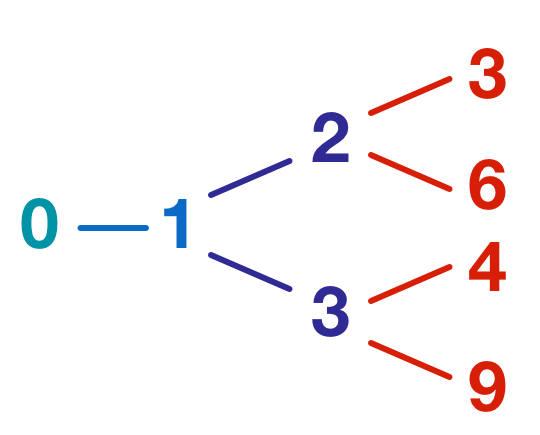# Add 1 Or Multiply By 3Consider the following game:

• Every move you can either add 1 or multiply by 3. Lines in the above diagram represent possible moves.

What is the smallest number that takes at least 15 moves to make?

Clarification: You need to find the smallest number that can be made in 15 moves but can't be made with any fewer than 15 moves. e.g. 15 could be made in 15 moves (add 1, 15 times) but it can also be made in 5 moves: $+1 \rightarrow \times 3 \rightarrow+1 \rightarrow+1 \rightarrow\times3,$

So, 15 would not be a valid answer.

×